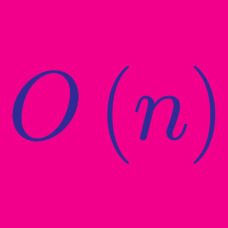Computer Science

# Big O Notation

Prefix sums of a sequence of numbers $a_0,a_1,a_2,a_3\ldots$ are given by a second sequence of numbers $s_0,s_1,s_2,s_3\ldots$ defined as

$s_j = \sum_{i=1}^{j}a_i$

The Python code below computes prefix sums.

 1 2 3 4 5 6 7 8 9 def s(a, j): prefix_sum = 0 while j >= 0: i = 0 while i <= j: prefix_sum += a[i] i += 1 j -= 1 return prefix_sum 

Analyze the the code and find the line or lines that will be executed the greatest number of times.

Select one or more

The fragment of Java code below describes a recursive method $P$ that takes two integer parameters $x$ and $n$ and returns an integer.

  1 2 3 4 5 6 7 8 9 10 11 12 13 public int P(int x , int n){ if (n == 0){ return 1; } if (n % 2 == 1){ int y = P(x, (n - 1) / 2); return x * y * y; } else{ int y = P(x, n / 2); return y * y; } } 

Analysis shows us that the running time of this function for large $n$ can be expressed as $T(n) = O(g(n))$ for some function $g(n)$. What is a valid function $g(n)$?

Details and assumptions

• $g(n)$ accurately describes the asymptotic runtime of $P(x,y)$.
• Disregard the limitations of the int primitive type and assume the behavior of the method is not affected for large inputs.
  1 2 3 4 5 6 7 8 9 10 11 int function(int n) { int i; if (n <= 0) { return 0; } else { i = random(n - 1); return function(i) + function(n - 1 - i); } } 

Consider the recursive algorithm above, where the random(int n) spends one unit of time to return a random integer which is evenly distributed within the range $[0,n]$. If the average processing time is $T(n)$, what is the value of $T(6)$?

Assume that all instructions other than the random cost a negligible amount of time.

The simple recursive python program below can be used to compute the $n^{th}$ Fibonnaci number.

 1 2 3 4 5 def Fibonacci(n): if n in [0, 1]: return n else: return Fibonacci(n-1) + Fibonacci(n-2) 

It can be shown that a tight bound of this program is given by $T(n) = \Theta(g(n))$ for some function $g(n)$. What family of functions is $g(n)$ in?

Details and assumptions

An obvious algorithm for exponentiation ($x^{n}$) takes $n-1$ multiplications. Suppose we propose a faster algorithm for the case where $n=2^{m}$. The "Big-O" complexity of such an algorithm can be expressed as:

$T(n)=O(g(n))$

Take the value of $g(n)$ for an arbitrary $n$. If we multiply $n$ by some constant $k$, how will the value of $g(n)$ most likely change?

×